## Exponential Functions

Exponential functions grow exponentially—that is, very, very quickly. Two squared is 4; 2 cubed is 8, but by the time you get to 2 7, you have, in four small steps from 8, already reached 128, and it only grows faster from there. Four more steps, for example, bring the value to 2,048.

Any function defined by y = b x , where b > 0, b ≠ 1, and x is a real number, is called an exponential function.

##### Example 1

Graph y = 2 x .

First find a sufficient number of ordered pairs to see the shape of the graph.

x

2 x = y

( x, y)

–3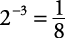–2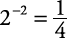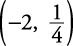–1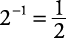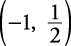0

20 = 1

(0, 1)

1

21 = 2

(1, 2)

2

22 = 4

(2, 4)

3

23 = 8

(3, 8)

Find these points and connect them to form a smooth curve. No value for x makes y become zero. The more negative x becomes, the smaller y becomes. The negative x‐axis becomes an asymptote for this function. The graph is shown in Figure 1.